International
Tables for
Crystallography
Volume B
Reciprocal space
Edited by U. Shmueli

International Tables for Crystallography (2006). Vol. B, ch. 1.3, p. 81   | 1 | 2 |

Section 1.3.4.3.5.4. Real symmetric transforms

G. Bricognea

aMRC Laboratory of Molecular Biology, Hills Road, Cambridge CB2 2QH, England, and LURE, Bâtiment 209D, Université Paris-Sud, 91405 Orsay, France

1.3.4.3.5.4. Real symmetric transforms

| top | pdf |

Conjugate symmetric (Section 1.3.2.4.2.3) implies that if the data X are real and symmetric [i.e.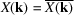and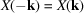], then so are the results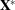. Thus ifcontains a centre of symmetry, F is real symmetric. There is no distinction (other than notation) between structure-factor and electron-density calculation; the algorithms will be described in terms of the former. It will be shown that if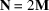, a real symmetric transform can be computed with only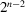partial transforms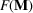instead of.

 (i) Decimation in time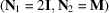Since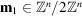we have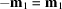and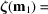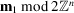. The decimated vectors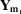are not only real, but have an internal symmetry expressed by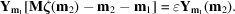This symmetry, however, is different for each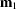so that we may multiplex two such vectors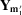and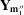into a general real vector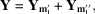for each of the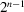pairs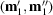. TheHermitian-symmetric transform vectors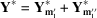can then be evaluated by the methods of Section 1.3.4.3.5.1(b)at the cost of onlygeneral complex. The demultiplexing relations by which the separate vectors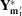and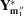may be recovered are most simply obtained by observing that the vectors Z after the twiddle-factor stage are real-valued since F(2I) has a real matrix. Thus, as in Section 1.3.4.3.5.1(c)(i),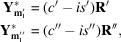where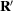and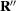are real vectors and where the multipliers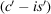and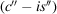are the inverse twiddle factors. Therefore,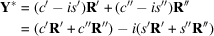and hence the demultiplexing relation for each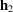: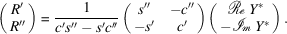The values of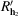and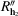at those pointswhere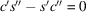can be evaluated directly while forming Y. This demultiplexing and the final stage of the calculation, namely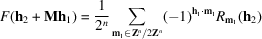need only be carried out for the unique half of the range of. (ii) Decimation in frequency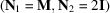Similarly, the vectors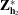of decimated and scrambled results are real and obey internal symmetries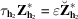which are different for each. For each of thepairs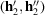the multiplexed vector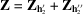is a Hermitian-symmetric vector without internal symmetry, and thereal vectors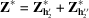may be evaluated at the cost of onlygeneral complexby the methods of Section 1.3.4.3.5.1(c). The individual transforms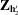and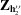may then be retrieved via the demultiplexing relations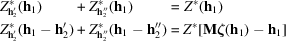which can be solved recursively as described in Section 1.3.4.3.5.1(b)(ii). This yields the unique half of the real symmetric results F.